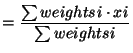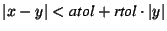IdentifiantMot de passe
Mot de passe oublié ?

Each of the operations discussed below returns an instance of numarray.ma class MaskedArray, having performed the desired operation element-wise. In most cases the array arguments can be masked arrays or numarray arrays or something that numarray can turn into a numarray array, such as a list of real numbers.

In most cases, if numarray has a function of the same name, the behavior of the one in numarray.ma is the same, except that it respects'' the mask.

## 18.5.1 Unary functions

The result of a unary operation will be masked wherever the original operand was masked. It may also be masked if the argument is not in the domain of the function. The following functions have their standard meaning:

absolute, arccos, arcsin, arctan, around, conjugate, cos, cosh, exp, floor, log, log10, negative (also as operator -), nonzero, sin, sinh, sqrt, tan, tanh.

 fabs( x)
The absolute value of x as a Float32 array.

## 18.5.2 Binary functions

Binary functions return a result that is masked wherever either of the operands were masked; it may also be masked where the arguments are not in the domain of the function.

add (also as operator +), subtract (also as operator -), multiply (also as operator *), divide (also as operator / ), power (also as operator **), remainder, fmod, hypot, arctan2, bitwise_and, bitwise_or, bitwise_xor.

## 18.5.3 Comparison operators

To compare arrays, use the following binary functions. Each of them returns a masked array of 1's and 0's.

equal, greater, greater_equal, less, less_equal, not_equal.

Note that as in numarray, you can use a scalar for one argument and an array for the other. Note: See section why operators and comparison functions are not excatly equivalent.

## 18.5.4 Logical operators

Arrays of logical values can be manipulated with:

logical_and, logical_not (unary), logical_or, logical_xor.

 alltrue( x)
Returns 1 if all elements of x are true. Masked elements are treated as true.

 sometrue( x)
Returns 1 if any element of x is true. Masked elements are treated as false.

## 18.5.5 Special array operators

 isarray( x)
Return true x is a masked array.

 rank( x)
The number of dimensions in x.

 shape( x)
Returns the shape of x, a tuple of array extents.

 resize( x, shape)
Returns a new array with specified shape.

 reshape( x, shape)
Returns a copy of x with the given new shape.

 ravel( x)

 concatenate( (a0, ... an), axis=0)
Concatenates the arrays a0, ... an along the specified axis.

 repeat( a, repeats, axis=0)
Repeat elements i of a repeats[i] times along axis. repeats is a sequence of length a.shape[axis] telling how many times to repeat each element.

 identity( n)
Returns the identity matrix of shape n by n.

 indices( dimensions, type=None)
Returns an array representing a grid of indices with row-only and column-only variation.

 len( x)
This is defined to be the length of the first dimension of x. This definition, peculiar from the array point of view, is required by the way Python implements slicing. Use size for the total length of x.

 size( x, axis=None)
This is the total size of x, or the length of a particular dimension axis whose index is given. When axis is given the dimension of the result is one less than the dimension of x.

 count( x, axis=None)
Count the number of (non-masked) elements in the array, or in the array along a certain axis. When axis is given the dimension of the result is one less than the dimension of x.

 arange( )
 arrayrange( )
 diagonal( )
 fromfunction( )
 ones( )
 zeros( )
are the same as in numarray, but return masked arrays.

 sum( )
 product( )
are called the same way as count; the difference is that the result is the sum or product of the unmasked element.

 average( x, axis=0, weights=None, returned=0)
Compute the average value of the non-masked elements of x along the selected axis. If weights is given, it must match the size and shape of x, and the value returned is:

 average(18.2)

In computing these sums, elements that correspond to those that are masked in x or weights are ignored. If successful a 2-tuple consisting of the average and the sum of the weights is returned.

 allclose( x, y, fill_value=1, rtol=1.e-5, atol=1.e-8)
Test whether or not arrays x and y are equal subject to the given relative and absolute tolerances. If fill_value is 1, masked values are considered equal, otherwise they are considered different. The formula used for elements where both x and y have a valid value is:(18.3)

This means essentially that both elements are small compared to atol or their difference divided by their value is small compared to rtol.

 allequal( x, y, fill_value=1)
This function is similar to allclose, except that exact equality is demanded. Note: Consider the problems of floating-point representations when using this function on non-integer numbers arrays.

 take( a, indices, axis=0)
Returns a selection of items from a. See the documentation of numarray.take in section 8.

 transpose( a, axes=None)
Performs a reordering of the axes depending on the tuple of indices axes; the default is to reverse the order of the axes.

 put( a, indices, values)
The opposite of take. The values of the array a at the locations specified in indices are set to the corresponding value of values. The array a must be a contiguous array. The argument indices can be any integer sequence object with values suitable for indexing into the flat form of a. The argument values must be any sequence of values that can be converted to the typecode of a.
>>> x = arange(6)
>>> put(x, [2,4], [20,40])
>>> print x
[ 0  1 20  3 40  5 ]

Note that the target array a is not required to be one-dimensional. Since it is contiguous and stored in row-major order, the array indices can be treated as indexing as elements in storage order.

The wrinkle on this for masked arrays is that if the locations being set by put are masked, the mask is cleared in those locations.

 choose( condition, t)
This function has a result shaped like condition. t must be a tuple. Each element of the tuple can be an array, a scalar, or the constant element masked (See section 18.6.1). Each element of the result is the corresponding element of t[i] where condition has the value i. The result is masked where condition is masked or where the selected element is masked or the selected element of t is the constant masked.

 where( condition, x, y)
Returns an array that is filled(x) where condition is true, filled(y) where the condition is false. One of x or y can be the constant element masked (See section 18.6.1). The result is masked where condition is masked, where the element selected from x or y is masked, or where x or y itself is the constant masked and it is selected.

 innerproduct( a, b)
 dot( a, b)
These functions work as in numarray, but missing values don't contribute. The result is always a masked array, possibly of length one, because of the possibility that one or more entries in it may be invalid since all the data contributing to that entry was invalid.

 outerproduct( a, b)
Produces a masked array such that result[i, j] = a[i] * b[j]. The result will be masked where a[i] or b[j] is masked.

 compress( condition, x, dimension=-1)
Compresses out only those valid values where condition is true. Masked values in condition are considered false.

 maximum( x, y=None)
 minimum( x, y=None)
Compute the maximum (minimum) valid values of x if y is None; with two arguments, they return the element-wise larger or smaller of valid values, and mask the result where either x or y is masked. If both arguments are scalars a scalar is returned.

 sort( x, axis=-1, value=None)
Returns the array x sorted along the given axis, with masked values treated as if they have a sort value of value but locations containing value are masked in the result if x had a mask to start with. Note: Thus if x contains value at a non-masked spot, but has other spots masked, the result may not be what you want.

 argsort( x, axis=-1, fill_value=None)
This function is unusual in that it returns a numarray array, equal to numarray.argsort(filled(x, fill_value), axis); this is an array of indices for sorting along a given axis.

## 18.5.6 Controlling the size of the string representations

 get_print_limit( )
 set_print_limit( n=0)
These functions are used to limit printing of large arrays; query and set the limit for converting arrays using str or repr.

If an array is printed that is larger than this, the values are not printed; rather you are informed of the type and size of the array. If n is zero, the standard numarray conversion functions are used.

When imported, numarray.ma sets this limit to 300, and the limit is also made to apply to standard numarray arrays as well.

Send comments to the NumArray community.